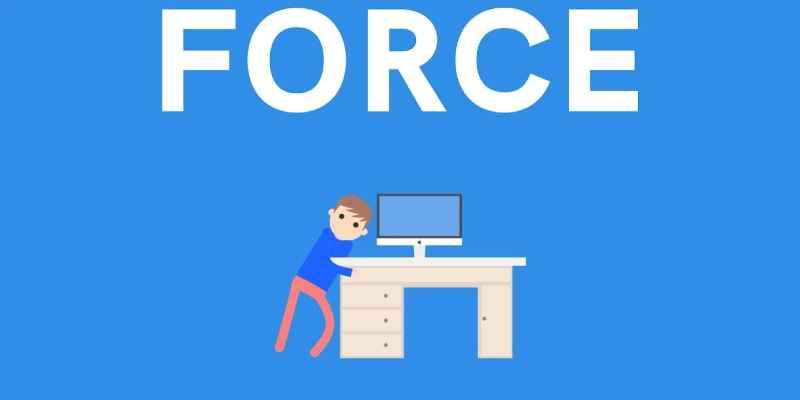### Trivia Quiz About Force And Types of ForceThe force on an object switches apropos to its mass and weight and size because the force has both magnitude and direction. It is a vector quantity. It shows the force due to its quantity. That it is also focused on a single point. Science says that force is a process of pulling and pushing, which changes its velocity according to its weight. Whenever an object is stopped or any object is moving on the road, it keeps moving straight till it does not move and does not stop until an external force is applied to it. Is the factor that changes the position of the object. Force equilibrium occurs when the resultant force acting on a point particle is zero (that is, the vector sum of all forces is zero). When dealing with an extended body, it is also necessary that the net torque be zero. Take this ultimate trivia quiz about Force.

#### 1. Complete the formula , Force = Mass * ______________.

 36103 Acceleration 36104 Velocity 36105 Weight 36106 Temperature

#### 2. Which type of force is caused by a large body like the planet Earth?

 36107 Tension 36108 Gravity 36109 Friction 36110 Nuclear

#### 3. A force can cause an object to ______________.

 36111 Accelerate 36112 Remain in place 36113 Change shape 36114 All of above

#### 4. Which type of force is exerted by an object trying to return to its natural length?

 36115 Gravity 36116 Nuclear 36117 Elastic 36118 Tension

#### 5. An object with a mass of 3 kg is accelerating at 2 m / s 2. What is the net force on the object?

 36119 3 N 36120 6 N 36121 9 N 36122 0 N

#### 6. The force that always acts opposite to the direction of the motion of an object is called.

 36123 Gravity 36124 Fraction 36125 Inertia 36126 Moment of inertia

#### 7. If an object is on a sloped surface of 45 degrees, in which direction is the normal force?

 36127 Straight up 36128 Straight down 36129 Parallel to the surface 36130 Perpendicular to the surface

#### 8. Which of these would not be considered an applied force?

 36131 Energy 36132 Pushing a chair 36133 Pulling a string 36134 None of these

#### 9. Balanced forces act on objects that are ___________.

 36135 Stationary 36136 In motion 36137 Rising or falling 36138 All of above

#### 10. Forces can change the.......

 36139 Slow down or speed up objects only 36140 Speed,direction or shape of an object 36141 Movement of objects but only if they were already in motion 36142 None of these

#### 11. What is the dimensional formula of force?

 36143 MLT^-1 36144 MLT^-2 36145 MLT^-3 36146 MLT^ 2

#### 12. A Newton is the international unit of measure for force. One newton is equal to....

 36147 10 kilogram meter per second squared 36148 1 kilogram meter per second squared 36149 64 kilogram meter per second squared 36150 100 kilogram meter per second squared